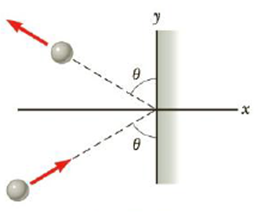Chapter 6, Problem 18P

Chapter
Section
Textbook Problem

A 3.00-kg steel ball strikes a massive wall at 10.0 m/s at an angle of θ = 60.0° with the plane of the wall. It bounces off the wall with the same speed and angle (Fig. P6.18). If the ball is in contact with the wall for 0.200 s, what is the average force exerted by the wall on the ball?Figure P6.18

To determine
Average force exerted by the wall on the ball.

Explanation

Given Info:The mass of the steel ball is 3.00kg , ball strikes the wall with velocity is 10.0m/s , angle 60° and duration of time that ball is in contact with wall is 0.20s .

The expression for x of average force is

(Fav)x=ΔpxΔt

• Fav is the average force along x axis
• Δpx is the change in momentum along x axis
• Δt is the time

The expression for y of average force is

(Fav)y=ΔpyΔt

• Fav is the average force along y axis
• Δpx is the change in momentum along y axis
• Δt is the time

Rewrite the above expression for x axis alone

(Fav)x=mΔt[(vx)f(vx)i](Fav)x=mΔt[vsinθ(+vsinθ)]

Rewrite the above expression for y axis alone

Still sussing out bartleby?

Check out a sample textbook solution.

See a sample solution

The Solution to Your Study Problems

Bartleby provides explanations to thousands of textbook problems written by our experts, many with advanced degrees!

Get Started

Absorption of the majority of nutrients takes place across the mucus-coated lining of the stomach. T F

Nutrition: Concepts and Controversies - Standalone book (MindTap Course List)

How are granules and super granules related? How do they differ?

Horizons: Exploring the Universe (MindTap Course List)

How many autosomes are present in a body cell of a human being? In a gamete?

Human Heredity: Principles and Issues (MindTap Course List)